Courses

# Test: Z-Transform Part - 2

## 10 Questions MCQ Test Signal and System | Test: Z-Transform Part - 2

Description
This mock test of Test: Z-Transform Part - 2 for Electrical Engineering (EE) helps you for every Electrical Engineering (EE) entrance exam. This contains 10 Multiple Choice Questions for Electrical Engineering (EE) Test: Z-Transform Part - 2 (mcq) to study with solutions a complete question bank. The solved questions answers in this Test: Z-Transform Part - 2 quiz give you a good mix of easy questions and tough questions. Electrical Engineering (EE) students definitely take this Test: Z-Transform Part - 2 exercise for a better result in the exam. You can find other Test: Z-Transform Part - 2 extra questions, long questions & short questions for Electrical Engineering (EE) on EduRev as well by searching above.
QUESTION: 1

### The unilateral z-transform of signal x[n] = u[ n + 4 ] is

Solution: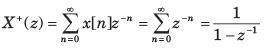QUESTION: 2

### The transfer function of a causal system is given as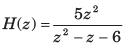The impulse response is

Solution: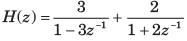h[n] is causal so ROC is |z|> 3,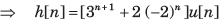QUESTION: 3

### A causal system has input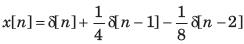and output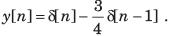The impulse response of this system is

Solution: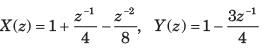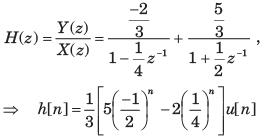QUESTION: 4

A causal system has input x[n] = (-3)n u[ n] and output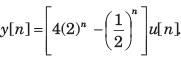The impulse response of this system is

Solution: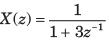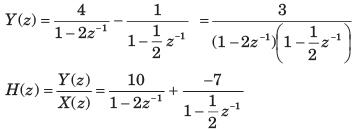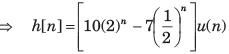QUESTION: 5

A system has impulse response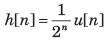The output y[n] to the input x[n] is given by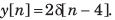The input x[n] is

Solution: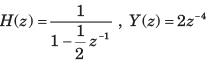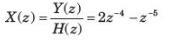=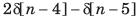QUESTION: 6

A system is described by the difference equation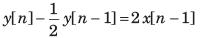The impulse response of the system is

Solution: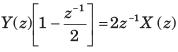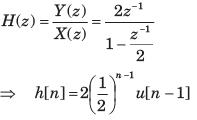QUESTION: 7

A system is described by the difference equation
y[n] = x[n]- x[n - 2] + x[n - 4] - x[n - 6]
The impulse response of system is

Solution: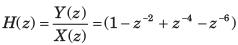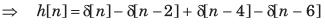QUESTION: 8

The impulse response of a system is given by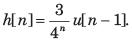The difference equation representation for this system is

Solution: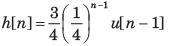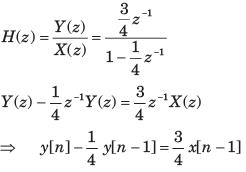QUESTION: 9

The impulse response of a system is given by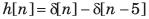The difference equation representation for this system is

Solution: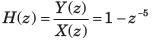⇒ y[n]= x[n] - x[n - 5 ]

QUESTION: 10

The transfer function of a system is given by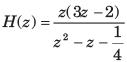The system is

Solution: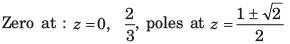(i) Not all poles are inside |z|= 1, the system is not causal and stable.
(ii) Not are poles and zero are inside |z|= 1, the system is not minimum phase.